## Figures index

#### S. Abdalla, F. Marzouki, S. Al-ameer, S. Turkestani

International Journal of Physics. 2013, 1(2), 41-48 doi:10.12691/ijp-1-2-3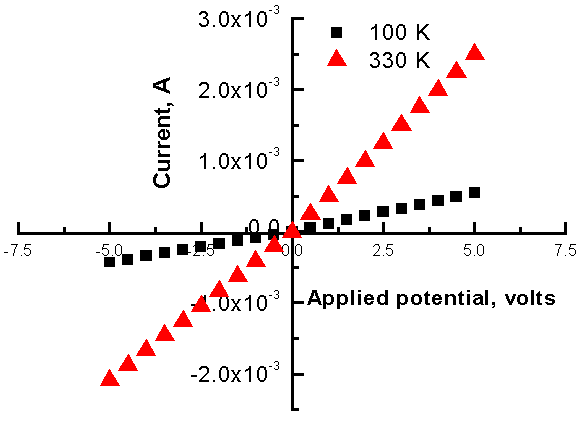• Figure 1. I –VT curves for n-type Al-GaN for different temperatures. The slope of these lines increases with temperature T and is directly proportional to the electrical conductivity σΩ1cm-1).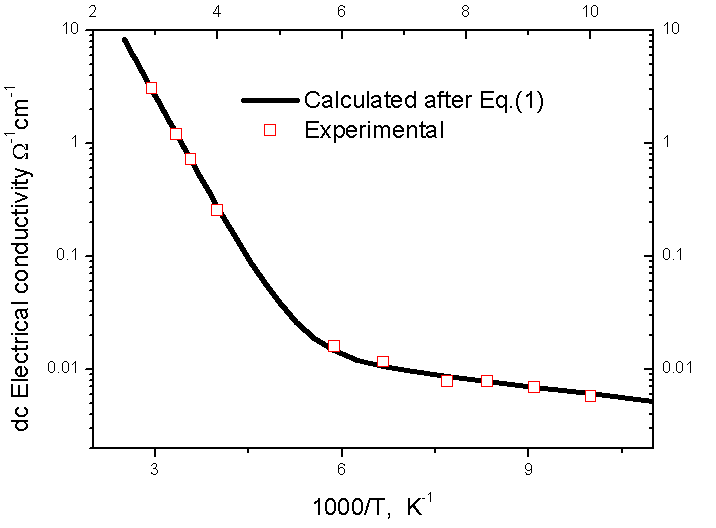• Figure 2. Electrical conductivity of n-GaN as a function of temperature: The solid line represents the calculated values after equation (1); it is calculated using Eq. (1) with 2 distinct activation energies at ΔE = 0.23 EV and 0.013EV while open squares designate the experimental values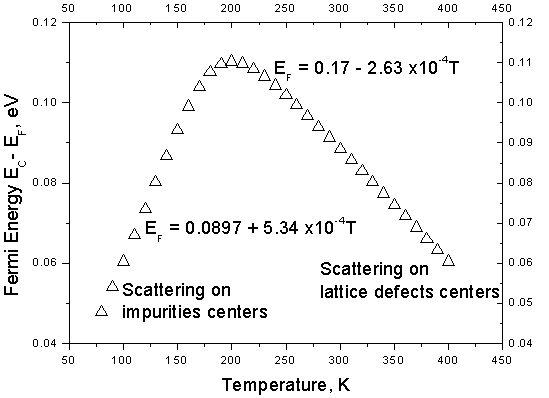• Figure 3. Fermi level energy as a function of temperature: at high temperature EF varies linearly as EF = 0.17 – 2.63 x10-4TeV and at low temperature EF varies linearly as EF = 0.0897 + 5.34 x10-4eV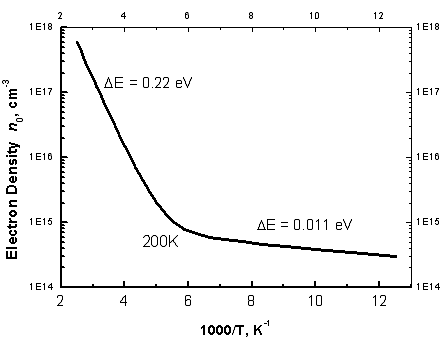• Figure 4. The electron density n0, calculated after Eq. (2) is presented as a function of temperature: the effect of both deep (EL1) and shallow (EL2) trapping levels are clearly shown at 0.22eV and 0.013eV respectively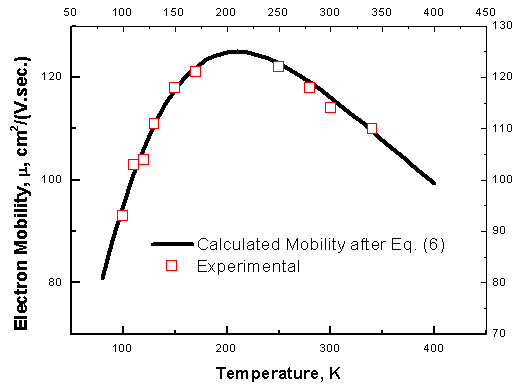• Figure 5. The electron mobility, μ as a function of temperature; two clear scattering mechanisms are present: (1) at low temperatures where μα T0.95 (scattering on impurity centers) and (2) at high temperatures where μα T-1.14(scattering on lattice defects)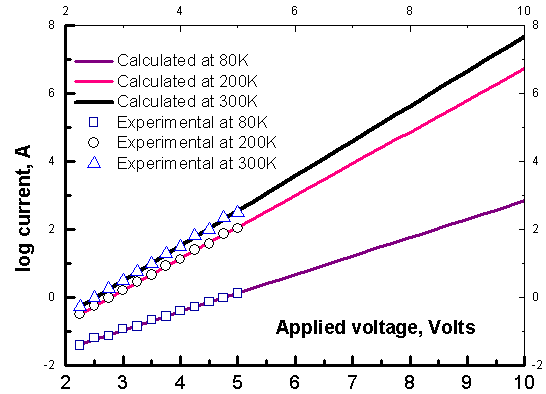• Figure 6. I – VT characteristics of Au-nGaN; solid lines represent calculated values after equation (7) at 80K and at 300K. Triangles symbolize the experimental values at 300K and squares denote the experimental values at 80K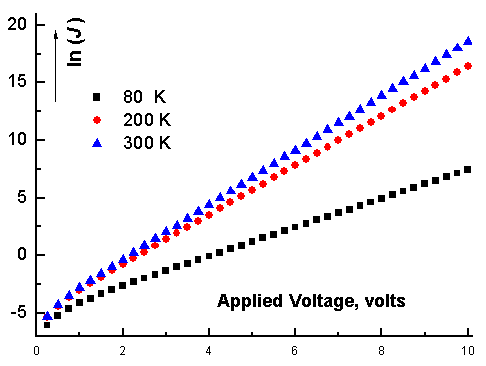• Figure 7. the natural logarithm of the current density ln(J) as a function of the applied potential Vappl for different temperatures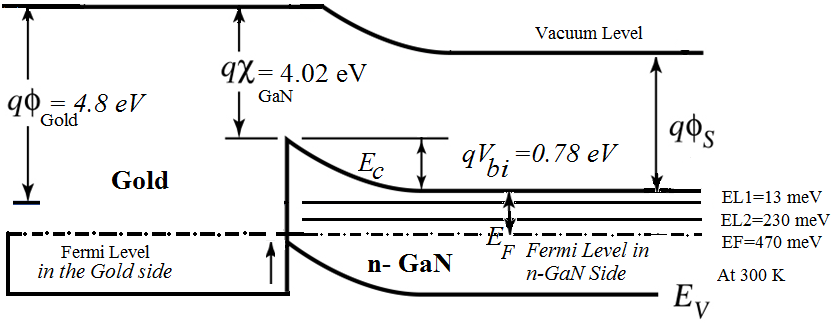• Figure 8. Energy band-diagram corresponding to room temperature for Au-n-GaN metal-semiconductor contact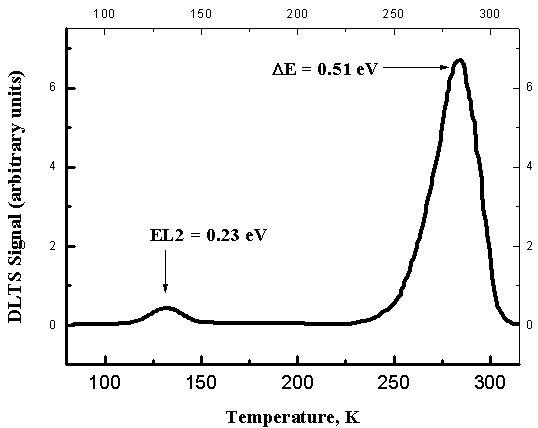• Figure 9. the Deep level transient spectroscopy signals as a function of temperature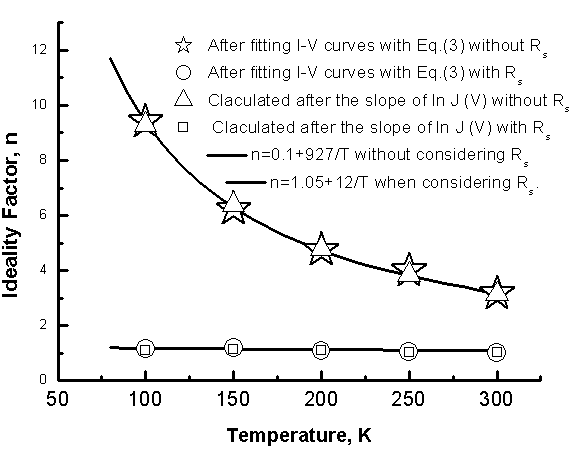• Figure 10. The temperature dependence of the ideality factor, n: Open triangles stand for values obtained after the slope of ln J curves when we neglecting the series resistance Rs and open stars represent n as fitting parameter of I-V curves with Eq. (7) when neglecting the presence of Rs. where Rs0 and α are constants: Rs0 = 21.18 Ω and α = 3.85 x 10-3K-1. This decaying behavior indicates that Rs has some semiconducting properties.